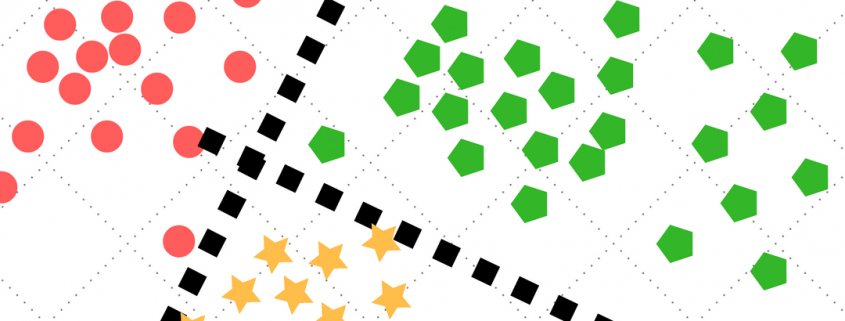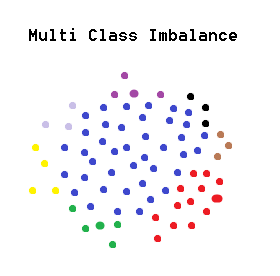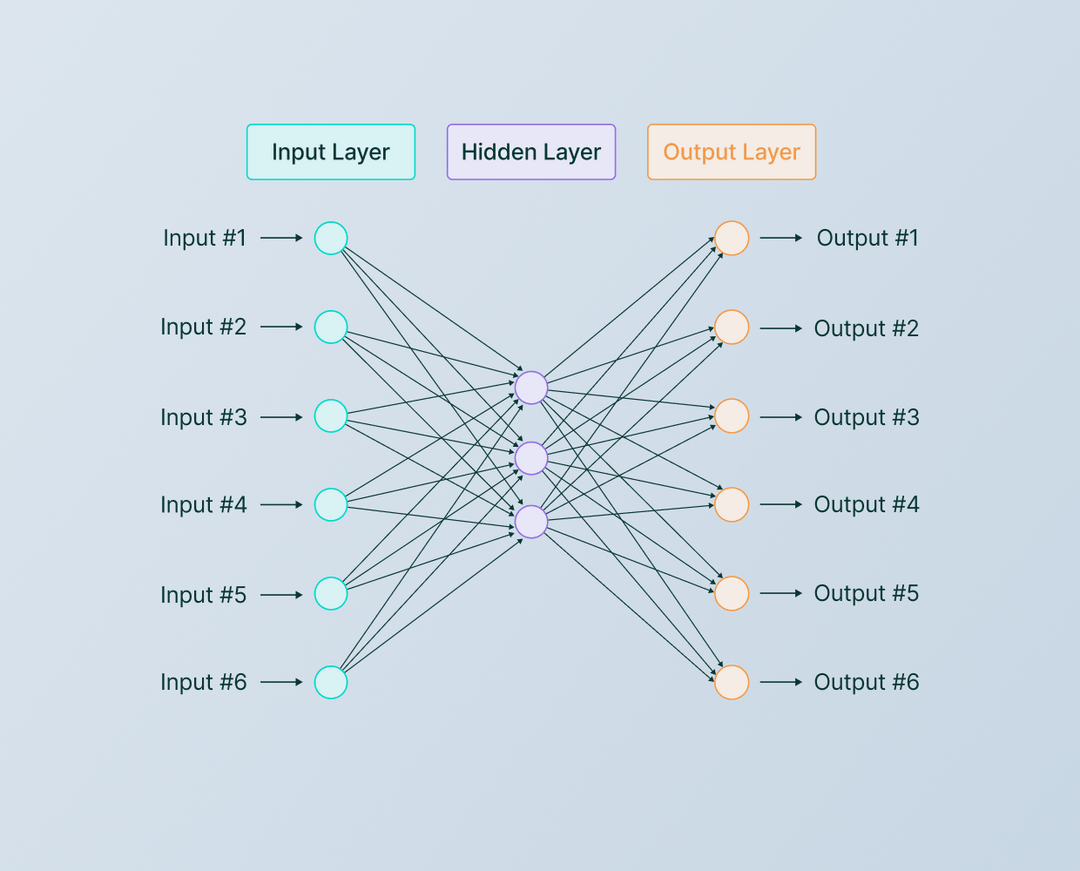# Multi-Class ClassificationIn machine learning, classification is the method of classifying data using certain input variables. A dataset with labels given (training dataset) is used to train the model in a way that the model can provide labels for datasets that are not yet labeled.

Under classification, there are 2 types of classifiers:

1. Binary Classification
2. Multi-Class Classification

Here let’s discuss Multiple Class classification in detail.

A classification problem where there are more than 2 classes amongst which the dataset is to be classified, for example: In the healthcare industry, it can be used to decide which disease a person has depended on the type of symptoms shown.

On websites like e-commerce websites like  Amazon, the products are classified under various categories ( Electronics, Furniture, etc.). Such classifications are not under the scope of human labor and can only be done using ML models.

Another use is in image classification; the most famous example for beginners is the recognition of a digit from the handwritten image dataset (MNIST Dataset). Here the images are classified into a number from 0 to 9.The most used Multiclass classification algorithms are:

1. Naive Bayes
2. Random Forest
3. KNN
4. Neural networks

## Imbalanced Dataset: A common problem

In an ideal condition, all the classes/ categories are equally represented in the dataset however, in the real-world scenario, this is never the case. This is often due to the unavailability of data and causes the dataset to be more biased towards one or more classes.

Let’s take the case of detecting a patient’s disease from symptoms. Dataset taken from a hospital might contain information on common diseases however there might be a lack of representation of the rarer diseases.

Such a case leads to the training of a biased model that causes miscalculations for minority classes in the dataset even though it has a high accuracy for majority classes.This problem can be solved by:

1. Collecting more data from various sources.
2. Using resampling techniques
1. Undersampling: Removing samples from the majority class
2. Oversampling: Adding more examples for the minority class
3. Creating synthetic Data: Certain deep learning techniques (like GANs) can be used to generate fake data to reduce the imbalance.

Working on multi-class classifiers:

## Binary Transform

As seen in the previous release, many ML models work solely on binary classification, so the Multiclass problem is broken into various binary classifications using one-vs-one or one-vs-rest strategies.

One Vs. Rest:

In this technique, one class is selected (target class) and classified against all the classes i.e., target class vs. remaining classes.

For example: In the case of product classification for a jewelry store’s e-commerce website where classes for products are Diamond, Gold, and Silver.

1. Classifier 1: Diamond vs. [Gold, Silver]
2. Classifier 2: Gold vs. [Diamond, Silver]
3. Classifier 3: Silver vs. [Diamond, Gold]

The binary classifier predicting the target class with the highest confidence is chosen and given as the final output.

One Vs. One:

In this technique, every pair of classes is classified against each other, i.e., one class vs. every other class individually.

This will lead to the creation of (N * (N-1))/2 classifiers where N is the total number of classes.

Taking the same example as above classifiers created are:

1. Classifier 1: Diamond vs. Gold
2. Classifier 2: Gold vs. Silver
3. Classifier 3; Diamond vs. Silver

After all, classifiers predict their respective classes the majority is taken as the final output.

This works best with SVM.

Don’t worry splitting into these classifiers and then getting the final output can easily be done with the help of Sklearn’s inbuilt functions.

This also explains why multi-class algorithms are more time and memory-consuming while training.

This seems like a tedious task which is simplified by the existence of Native multiclass algorithms.

Algorithms such as K-Nearest Neighbours, Naive Bayes, and Decision trees are created in a way that they can take care of multiclass problems without requiring any extra strategies.

Naive Bayes works with the Bayes theorem and assumes all classes and features are independent. It works well with large datasets with categorical features.

Decision trees take the entire dataset in the root node and progressively split it into a tree-like structure until it reaches the leaf nodes providing the final output. This works with categorical and continuous datasets and has a relatively low training time.

Example of a Decision tree classifier

K-nearest neighbor is a classification technique that does not require training and finds the ‘k’ nearest data points to the given unknown data point. The class present in most of these k points is taken as the output.

The latest way to tackle multi-class classification is using neural networks where the algorithm simulates the human brain and has small units called notes for the computation of the dataset.These are certain ways by which multi-class classification is done. More in-depth explanations for each algorithm will be in future releases.

Multi-class classification is a fundamental problem in machine learning where the task is to classify instances into one of multiple mutually exclusive classes. In this article, we will explore various strategies and techniques for multi-class classification, including multinomial logistic regression, evaluation metrics, handling imbalanced data, error analysis, techniques for improving performance, and real-world case studies.

### I. Multinomial Logistic Regression for Multi-Class Classification

Multinomial logistic regression is an extension of binary logistic regression to handle multi-class classification problems. Instead of treating each class separately, multinomial logistic regression models the probabilities of each class directly. It uses the softmax function to normalize the predicted probabilities across all classes.

### II. Evaluation Metrics for Multi-Class Classification

Evaluating the performance of a multi-class classification model requires appropriate metrics. Common evaluation metrics include accuracy, precision, recall, F1-score, and the confusion matrix. These metrics provide insights into the model’s ability to correctly classify instances across multiple classes.

### III. Handling Imbalanced Data in Multi-Class Classification

Imbalanced data, where the number of instances in different classes is uneven, is a common challenge in multi-class classification. Techniques such as over-sampling, under-sampling, and data augmentation can be employed to address class imbalance and improve model performance.

### IV. Error Analysis in Multi-Class Classification

Error analysis helps understand the patterns and sources of misclassifications in multi-class classification. By analyzing misclassified instances, we can gain insights into the specific challenges faced by the model and identify areas for improvement.

### V. Techniques for Improving Multi-Class Classification Performance

Several techniques can enhance the performance of multi-class classification models. These include feature engineering, dimensionality reduction, ensemble methods (e.g., random forests, gradient boosting), and neural network architectures (e.g., deep learning models).

### VI. Case Studies: Applications of Multi-Class Classification

Real-world case studies showcase the practical application of multi-class classification. For example, in the field of image recognition, multi-class classification is used to classify objects, identify handwritten digits, and detect diseases from medical images. Other applications include text categorization, sentiment analysis, and speech recognition.

In conclusion, multi-class classification is a challenging task with diverse strategies and techniques. Understanding multinomial logistic regression, evaluation metrics, handling imbalanced data, error analysis, techniques for performance improvement, and real-world case studies is crucial for effectively addressing multi-class classification problems. By leveraging these approaches, practitioners can develop accurate and robust multi-class classification models across various domains.